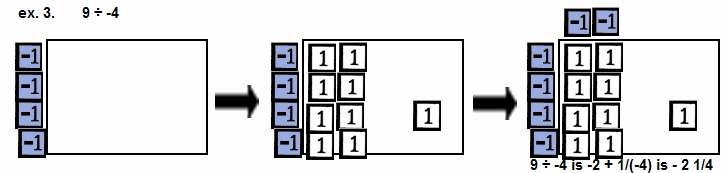The Visual / Auditory / Symbolic / Kinesthetic Approach to Algebra

 Multiplication & Division

 Multiplication

 To multiply by a negative, change the sign and multiply the size. To multiply by a positive multiply the size.Multiply.
 1st: Use a blank rectangular sheet of paper to separate the storage region from the display area and to create a border for the rectangular area created.
 2nd: Represent one factor (usually the fist) vertically in the storage on the left edge of the display area. The factor acts as a measure of the length of the side.
 3rd: Represent the other factor horizontally in the storage on the top edge of the display area. The factor acts as a measure of the length of the side.
 4th: Multiply a top tile by a side tile filling in the corresponding region of the display area with the required tile. These tiles represent the product and the area of the region.
 5th: The product is produced in the display area.Two-Digit Multiplication
 ex. 9. Multiply.In the basic set of Term Tiles, a 10-tile is not included. If it were, this is what the multiplication fo 2 times 13 would look like.     Two-digit multiplication is included here because it foreshadows algebraic multiplication involving binomials and other polynomials.

 ex. 10. Multiply.In the basic set of Term Tiles, a 10-tile, -10-tile, 100-tile and -100-tile do not exist.     If these tiles were included this is what the representaion and product would look like.     It is NOT necessary to use tiles -- a graphic is sufficient.

 Division

 To divide by a negative, change the sign and divided as usual. To divide by a positive, divide as usual.Divide.
 1st: Use a blank rectangular sheet of paper to separate the storage region from the display area and to create a border for the rectangular area created.
 2nd: Represent the divisor vertically in storage on the left edge of the display area.
 3rd: Begin with the terms (tiles) of highest degree placing tiles in the upper left corner first.
 4th: Represent the dividend in rows in the display area matching the divisor. The remainder will, of course, be extra.
 5th: Represent the appropriate quotient horizontally in the storage area as dictated by the dividend.
 6th: Read the quotient and the remainder.www.termtiles.com, Unit 15   © 2008, A. Azzolino